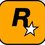# Probability Challenge!

$$A_{i};i=1,2,3..n$$ are $$n$$ persons which play a game of tossing a coin. The game starts when $$A_{1}$$ tosses the coin. If he gets a tail, the next person i.e. $$A_{2}$$ gets a chance to toss the coin and so on. Whosoever gets a head first wins the game. If no one wins, the game is played again. Find the probability of event in which the $${r}^{th}$$ person i.e. $$A_{r}$$ wins the game.Note by Rohit Ner
5 years, 9 months ago

This discussion board is a place to discuss our Daily Challenges and the math and science related to those challenges. Explanations are more than just a solution — they should explain the steps and thinking strategies that you used to obtain the solution. Comments should further the discussion of math and science.

When posting on Brilliant:

• Use the emojis to react to an explanation, whether you're congratulating a job well done , or just really confused .
• Ask specific questions about the challenge or the steps in somebody's explanation. Well-posed questions can add a lot to the discussion, but posting "I don't understand!" doesn't help anyone.
• Try to contribute something new to the discussion, whether it is an extension, generalization or other idea related to the challenge.

MarkdownAppears as
*italics* or _italics_ italics
**bold** or __bold__ bold
- bulleted- list
• bulleted
• list
1. numbered2. list
1. numbered
2. list
Note: you must add a full line of space before and after lists for them to show up correctly
paragraph 1paragraph 2

paragraph 1

paragraph 2

[example link](https://brilliant.org)example link
> This is a quote
This is a quote
    # I indented these lines
# 4 spaces, and now they show
# up as a code block.

print "hello world"
# I indented these lines
# 4 spaces, and now they show
# up as a code block.

print "hello world"
MathAppears as
Remember to wrap math in $$ ... $$ or $ ... $ to ensure proper formatting.
2 \times 3 $2 \times 3$
2^{34} $2^{34}$
a_{i-1} $a_{i-1}$
\frac{2}{3} $\frac{2}{3}$
\sqrt{2} $\sqrt{2}$
\sum_{i=1}^3 $\sum_{i=1}^3$
\sin \theta $\sin \theta$
\boxed{123} $\boxed{123}$

Sort by:

The probability that the game is played again is $\dfrac{1}{2^{n}}$.The probability that the r th person wins in the x th round of the game is $\dfrac{1}{2^{xn+r}}$.Hence the answer,according to me should be,$\sum_{x=1}^{\infty}\dfrac{1}{2^{xn+r}}$. This is a simple G.P. What do you think?

- 5 years, 9 months ago

oh yeah! i too got the same answer! cheers!

- 5 years, 9 months ago

Cheers!From where did you get this question?

- 5 years, 9 months ago

Actually the question struck to my mind when my sir was teaching us conditional probability.its a generalized version I managed to frame. :P

- 5 years, 9 months ago

OHk!

- 5 years, 9 months ago